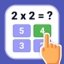# Multiplication Table Android

1.5.1

Are you learning the Multiplication Table or would you like to help your children study? Learn, play, and practice with the multiplication table app

## Learn the multiplication tables

June 5, 2023
7 / 10

Multiplication Table Android is an interesting educational app to study mathematics. Although the main idea is that users can learn and review the multiplication tables, it actually offers much more than that, as it also includes review exercises and several games.

### Study, learn, play and practice

Studying math can be a lot of fun with the right approach. And that is what this app shows, which starts from a minimalist base to provide a complete didactic experience. The contents of Multiplication Table APK are divided into four sections:

• Puzzles. Here we will find different mini-games of mathematics, logic and observation.
• Training. This section serves to develop our math skills, being able to train with addition, subtraction, multiplication and division.
• Multiplication tables. In this section there are the multiplication tables from 1 to 30. In addition, it also has practical exercises to reinforce the knowledge learned.
• Tests. Math tests with three levels of difficulty.

Learn the multiplication table from 1 to 20 and then test your knowledge by solving the multiplication and division examples.

In short, this platform contains everything you need to study math, review, practice, learn and even have fun. And best of all, you can download Multiplication Table for free.

• Minimum operating system requirements: Android 5.0.
• Offers in-app purchases.

Features

• June 5, 2023

• 37 MB

• free
• Android

• de.softan.multiplication.table